Documentation

Bayesian Stochastic Search Variable Selection

This example shows how to implement stochastic search variable selection (SSVS), a Bayesian variable selection technique for linear regression models.

Introduction

Consider this Bayesian linear regression model.

${y}_{t}=\sum _{k}{\beta }_{k}{x}_{tk}+{\epsilon }_{t}.$

• The regression coefficients ${\beta }_{k}|{\sigma }^{2}\sim N\left({\mu }_{j},{\sigma }^{2}{V}_{k}\right)$.

• $k=0,...,p$.

• The disturbances ${\epsilon }_{t}\sim N\left(0,{\sigma }^{2}\right)$.

• The disturbance variance ${\sigma }^{2}\sim IG\left(A,B\right)$, where $IG\left(A,B\right)$ is the inverse gamma distribution with shape A and scale B.

The goal of variable selection is to include only those predictors supported by data in the final regression model. One way to do this is to analyze the ${2}^{p}$ permutations of models, called regimes, where models differ by the coefficients that are included. If $p$ is small, then you can fit all permutations of models to the data, and then compare the models by using performance measures, such as goodness-of-fit (for example, Akaike information criterion) or forecast mean squared error (MSE). However, for even moderate values of $p$, estimating all permutations of models is inefficient.

A Bayesian view of variable selection is a coefficient, being excluded from a model, has a degenerate posterior distribution. That is, the excluded coefficient has a Dirac delta distribution, which has its probability mass concentrated on zero. To circumvent the complexity induced by degenerate variates, the prior for a coefficient being excluded is a Gaussian distribution with a mean of 0 and a small variance, for example $N\left(0,0.{1}^{2}\right)$. Because the prior is concentrated around zero, the posterior must also be concentrated around zero.

The prior for the coefficient being included can be $N\left(\mu ,V\right)$, where $V$ is sufficiently away from zero and $\mu$ is usually zero. This framework implies that the prior of each coefficient is a Gaussian mixture model.

Consider the latent, binary random variables ${\gamma }_{k}$, $k=0,...,p$, such that:

• ${\gamma }_{k}=1$ indicates that ${\beta }_{k}\sim N\left(0,{\sigma }^{2}{V}_{1k}\right)$ and that ${\beta }_{k}$ is included in the model.

• ${\gamma }_{k}=0$ indicates that ${\beta }_{k}\sim N\left(0,{\sigma }^{2}{V}_{2k}\right)$ and that ${\beta }_{k}$ is excluded from the model.

• ${\gamma }_{k}\sim Bernoulli\left({g}_{k}\right)$.

• The sample space of ${\gamma }_{k}$ has a cardinality of ${2}^{\mathit{p}+1}$, and each element is a $p+1$-D vector of zeros or ones.

• ${V}_{2k}$ is a small, positive number and ${V}_{1k}>{V}_{2k}$.

• Coefficients ${\beta }_{j}$ and ${\beta }_{k}$, $j\ne k$ are independent, a priori.

One goal of SSVS is to estimate the posterior regime probabilities ${g}_{k}$, the estimates that determine whether corresponding coefficients should be included in the model. Given ${\beta }_{k}$, ${\gamma }_{k}$ is conditionally independent of the data. Therefore, for $k=0,...,p$, this equation represents the full conditional posterior distribution of the probability that variable k is included in the model:

$P\left({\gamma }_{k}=1|\beta ,{\sigma }^{2},{\gamma }_{\ne k}\right)\propto {g}_{k}\varphi \left({\beta }_{k};0,{\sigma }^{2}{V}_{1k}\right),$

where $\varphi \left(\mu ,{\sigma }^{2}\right)$ is the pdf of the Gaussian distribution with scalar mean $\mu$ and variance ${\sigma }^{2}$.

Econometrics Toolbox™ has two Bayesian linear regression models that specify the prior distributions for SSVS: mixconjugateblm and mixsemiconjugateblm. The framework presented earlier describes the priors of the mixconjugateblm model. The difference between the models is that $\beta$ and ${\sigma }^{2}$ are independent, a priori, for mixsemiconjugateblm models. Therefore, the prior variance of ${\beta }_{k}$ is ${V}_{k1}$ (${\gamma }_{\mathit{k}}=1$) or ${V}_{k2}$ (${\gamma }_{\mathit{k}}=0$).

After you decide which prior model to use, call bayeslm to create the model and specify hyperparameter values. Supported hyperparameters include:

• Intercept, a logical scalar specifying whether to include an intercept in the model.

• Mu, a (p + 1)-by-2 matrix specifying the prior Gaussian mixture means of $\beta$. The first column contains the means for the component corresponding to ${\gamma }_{k}=1$, and the second column contains the means corresponding to ${\gamma }_{k}=0$. By default, all means are 0, which specifies implementing SSVS.

• V, a (p + 1)-by-2 matrix specifying the prior Gaussian mixture variance factors (or variances) of $\beta$. Columns correspond to the columns of Mu. By default, the variance of the first component is 10 and the variance of the second component is 0.1.

• Correlation, a (p + 1)-by-(p + 1) positive definite matrix specifying the prior correlation matrix of $\beta$ for both components. The default is the identity matrix, which implies that the regression coefficients are uncorrelated, a priori.

• Probability, a (p + 1)-D vector of prior probabilities of variable inclusion (${g}_{k}$, k = 0,...,_p_) or a function handle to a custom function. ${\gamma }_{j}$ and ${\gamma }_{k}$, $j\ne k$, are independent, a priori. However, using a function handle (@functionname), you can supply a custom prior distribution that specifies dependencies between ${\gamma }_{j}$ and ${\gamma }_{k}$. For example, you can specify forcing ${x}_{2}$ out of the model if ${x}_{4}$ is included.

After you create a model, pass it and the data to estimate. The estimate function uses a Gibbs sampler to sample from the full conditionals, and estimate characteristics of the posterior distributions of $\beta$ and ${\sigma }^{2}$. Also, estimate returns posterior estimates of ${g}_{k}$.

For this example, consider creating a predictive linear model for the US unemployment rate. You want a model that generalizes well. In other words, you want to minimize the model complexity by removing all redundant predictors and all predictors that are uncorrelated with the unemployment rate.

Load and Preprocess Data

Load the US macroeconomic data set Data_USEconModel.mat.

The data set includes the MATLAB® timetable DataTable, which contains 14 variables measured from Q1 1947 through Q1 2009; UNRATE is the US unemployment rate. For more details, enter Description at the command line.

Plot all series in the same figure, but in separate subplots.

figure;
for j = 1:size(DataTable,2)
subplot(4,4,j)
plot(DataTable.Time,DataTable{:,j});
title(DataTable.Properties.VariableNames(j));
end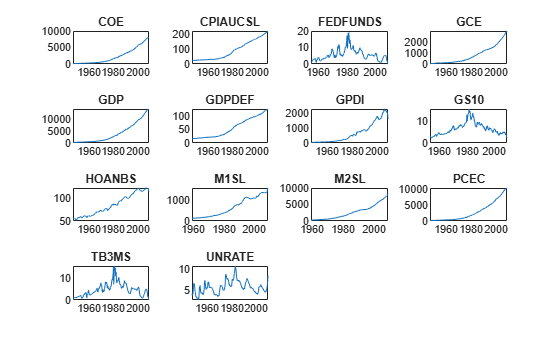All series except FEDFUNDS, GS10, TB3MS, and UNRATE appear to have an exponential trend.

Apply the log transform to those variables with an exponential trend.

hasexpotrend = ~ismember(DataTable.Properties.VariableNames,...
["FEDFUNDS" "GD10" "TB3MS" "UNRATE"]);
DataTableLog = varfun(@log,DataTable,'InputVariables',...
DataTable.Properties.VariableNames(hasexpotrend));
DataTableLog = [DataTableLog DataTable(:,DataTable.Properties.VariableNames(~hasexpotrend))];

DataTableLog is a timetable like DataTable, but those variables with an exponential trend are on the log scale.

Coefficients that have relatively large magnitudes tend to dominate the penalty in the lasso regression objective function. Therefore, it is important that variables have a similar scale when you implement lasso regression. Compare the scales of the variables in DataTableLog by plotting their box plots on the same axis.

figure;
boxplot(DataTableLog.Variables,'Labels',DataTableLog.Properties.VariableNames);
h = gcf;
h.Position(3) = h.Position(3)*2.5;
title('Variable Box Plots');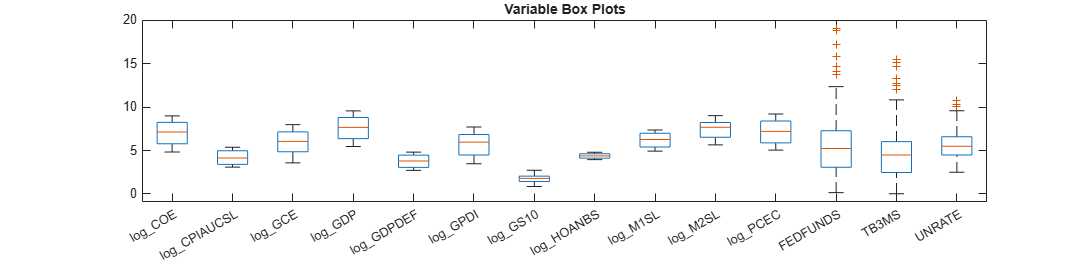The variables have fairly similar scales.

To tune the prior Gaussian mixture variance factors, follow this procedure:

1. Partition the data into estimation and forecast samples.

2. Fit the models to the estimation sample and specify, for all $k$, ${V}_{1k}=\left\{10,\phantom{\rule{0.2777777777777778em}{0ex}}50,\phantom{\rule{0.2777777777777778em}{0ex}}100\right\}$ and ${V}_{2k}=\left\{0.05,\phantom{\rule{0.2777777777777778em}{0ex}}0.1,\phantom{\rule{0.2777777777777778em}{0ex}}0.5\right\}$.

3. Use the fitted models to forecast responses into the forecast horizon.

4. Estimate the forecast MSE for each model.

5. Choose the model with the lowest forecast MSE.

George and McCulloch suggest another way to tune the prior variances of $\beta$ in .

Create estimation and forecast sample variables for the response and predictor data. Specify a forecast horizon of 4 years (16 quarters).

fh = 16;
y = DataTableLog.UNRATE(1:(end - fh));
yF = DataTableLog.UNRATE((end - fh + 1):end);
isresponse = DataTable.Properties.VariableNames == "UNRATE";
X = DataTableLog{1:(end - fh),~isresponse};
XF = DataTableLog{(end - fh + 1):end,~isresponse};
p = size(X,2); % Number of predictors
predictornames = DataTableLog.Properties.VariableNames(~isresponse);

Create Prior Bayesian Linear Regression Models

Create prior Bayesian linear regression models for SSVS by calling bayeslm and specifying the number of predictors, model type, predictor names, and component variance factors. Assume that $\beta$ and ${\sigma }^{2}$ are dependent, a priori (mixconjugateblm model).

V1 = [10 50 100];
V2 = [0.05 0.1 0.5];
numv1 = numel(V1);
numv2 = numel(V2);

PriorMdl = cell(numv1,numv2); % Preallocate

for k = 1:numv2
for j = 1:numv1
V = [V1(j)*ones(p + 1,1) V2(k)*ones(p + 1,1)];
PriorMdl{j,k} = bayeslm(p,'ModelType','mixconjugateblm',...
'VarNames',predictornames,'V',V);
end
end

PriorMdl is a 3-by-3 cell array, and each cell contains a mixconjugateblm model object.

Plot the prior distribution of log_GDP for the models in which V2 is 0.5.

for j = 1:numv1
[~,~,~,h] = plot(PriorMdl{j,3},'VarNames',"log_GDP");
title(sprintf("Log GDP, V1 = %g, V2 = %g",V1(j),V2(3)));
h.Tag = strcat("fig",num2str(V1(j)),num2str(V2(3)));
end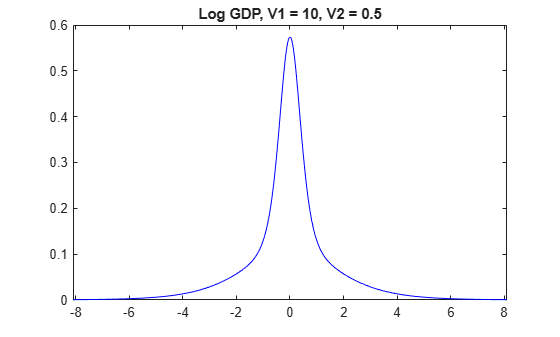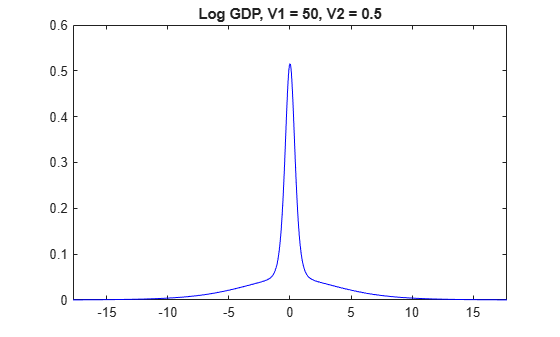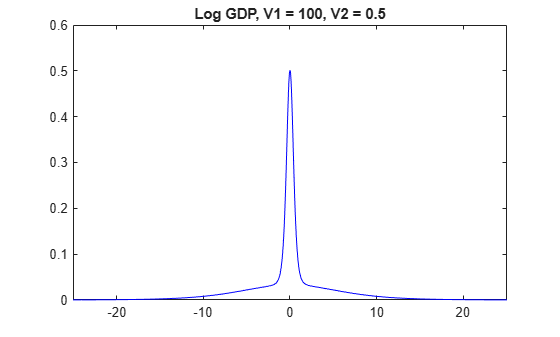The prior distributions of $\beta$ have the spike-and-slab shape. When V1 is low, more of the distribution is concentrated around 0, which makes it more difficult for the algorithm to attribute a high value for beta. However, variables the algorithm identifies as important are regularized, in that the algorithm does not attribute a high magnitude to the corresponding coefficients.

When V1 is high, more density occurs well away from zero, which makes it easier for the algorithm to attribute non-zero coefficients to important predictors. However, if V1 is too high, then important predictors can have inflated coefficients.

Perform SSVS Variable Selection

To perform SSVS, estimate the posterior distributions by using estimate. Use the default options for the Gibbs sampler.

PosteriorMdl = cell(numv1,numv2);
PosteriorSummary = cell(numv1,numv2);

rng(1); % For reproducibility
for k = 1:numv2
for j = 1:numv1
[PosteriorMdl{j,k},PosteriorSummary{j,k}] = estimate(PriorMdl{j,k},X,y,...
'Display',false);
end
end

Each cell in PosteriorMdl contains an empiricalblm model object storing the full conditional posterior draws from the Gibbs sampler. Each cell in PosteriorSummary contains a table of posterior estimates. The Regime table variable represents the posterior probability of variable inclusion (${g}_{k}$).

Display a table of posterior estimates of ${g}_{k}$.

RegimeTbl = table(zeros(p + 2,1),'RowNames',PosteriorSummary{1}.Properties.RowNames);
for k = 1:numv2
for j = 1:numv1
vname = strcat("V1_",num2str(V1(j)),"__","V2_",num2str(V2(k)));
vname = replace(vname,".","p");
tmp = table(PosteriorSummary{j,k}.Regime,'VariableNames',vname);
RegimeTbl = [RegimeTbl tmp];
end
end
RegimeTbl.Var1 = [];
RegimeTbl
RegimeTbl=15×9 table
V1_10__V2_0p05    V1_50__V2_0p05    V1_100__V2_0p05    V1_10__V2_0p1    V1_50__V2_0p1    V1_100__V2_0p1    V1_10__V2_0p5    V1_50__V2_0p5    V1_100__V2_0p5
______________    ______________    _______________    _____________    _____________    ______________    _____________    _____________    ______________

Intercept           0.9692                 1                 1            0.9501                1                 1           0.9487           0.9999                 1
log_COE             0.4686            0.4586            0.5102            0.4487           0.3919            0.4785           0.4575           0.4147            0.4284
log_CPIAUCSL        0.9713            0.3713            0.4088             0.971           0.3698            0.3856            0.962           0.3714            0.3456
log_GCE             0.9999                 1                 1            0.9978                1                 1           0.9959                1                 1
log_GDP             0.7895            0.9921            0.9982            0.7859           0.9959                 1           0.7908           0.9975            0.9999
log_GDPDEF          0.9977                 1                 1                 1                1                 1           0.9996                1                 1
log_GPDI                 1                 1                 1                 1                1                 1                1                1                 1
log_GS10                 1                 1            0.9991                 1                1            0.9992                1           0.9992             0.994
log_HOANBS          0.9996                 1                 1            0.9887                1                 1           0.9763                1                 1
log_M1SL                 1                 1                 1                 1                1                 1                1                1                 1
log_M2SL            0.9989            0.9993            0.9913            0.9996           0.9998            0.9754           0.9951           0.9983            0.9856
log_PCEC            0.4457            0.6366            0.8421            0.4435           0.6226            0.8342           0.4614            0.624              0.85
FEDFUNDS            0.0762            0.0386            0.0237            0.0951           0.0465            0.0343           0.1856           0.0953             0.068
TB3MS               0.2473            0.1788            0.1467            0.2014           0.1338            0.1095           0.2234           0.1185            0.0909
Sigma2                 NaN               NaN               NaN               NaN              NaN               NaN              NaN              NaN               NaN

Using an arbitrary threshold of 0.10, all models agree that FEDFUNDS is an insignificant or redundant predictor. When V1 is high, TB3MS borders on being insignificant.

Forecast responses and compute forecast MSEs using the estimated models.

yhat = zeros(fh,numv1*numv2);
fmse = zeros(numv1,numv2);

for k = 1:numv2
for j = 1:numv1
idx = ((k - 1)*numv1 + j);
yhat(:,idx) = forecast(PosteriorMdl{j,k},XF);
fmse(j,k) = sqrt(mean((yF - yhat(:,idx)).^2));
end
end

Identify the variance factor settings that yield the minimum forecast MSE.

minfmse = min(fmse,[],'all');
[idxminr,idxminc] = find(abs(minfmse - fmse) < eps);
bestv1 = V1(idxminr)
bestv1 = 100
bestv2 = V2(idxminc)
bestv2 = 0.0500

Estimate an SSVS model using the entire data set and the variance factor settings that yield the minimum forecast MSE.

XFull = [X; XF];
yFull = [y; yF];
EstMdl = estimate(PriorMdl{idxminr,idxminc},XFull,yFull);
Method: MCMC sampling with 10000 draws
Number of observations: 201
Number of predictors:   14

|   Mean      Std          CI95         Positive  Distribution  Regime
-------------------------------------------------------------------------------------
Intercept    |  29.4598  4.2723   [21.105, 37.839]     1.000     Empirical    1
log_COE      |   3.5380  3.0180   [-0.216,  9.426]     0.862     Empirical   0.7418
log_CPIAUCSL |  -0.6333  1.7689   [-5.468,  2.144]     0.405     Empirical   0.3711
log_GCE      |  -9.3924  1.4699  [-12.191, -6.494]     0.000     Empirical    1
log_GDP      |  16.5111  3.7131   [ 9.326, 23.707]     1.000     Empirical    1
log_GDPDEF   |  13.0146  2.3992   [ 9.171, 19.131]     1.000     Empirical    1
log_GPDI     |  -5.9537  0.6083   [-7.140, -4.756]     0.000     Empirical    1
log_GS10     |   1.4485  0.3852   [ 0.680,  2.169]     0.999     Empirical   0.9868
log_HOANBS   | -16.0240  1.5361  [-19.026, -13.048]    0.000     Empirical    1
log_M1SL     |  -4.6509  0.6815   [-5.996, -3.313]     0.000     Empirical    1
log_M2SL     |   5.3320  1.3003   [ 2.738,  7.770]     0.999     Empirical   0.9971
log_PCEC     |  -9.9025  3.3904  [-16.315, -2.648]     0.006     Empirical   0.9858
FEDFUNDS     |  -0.0176  0.0567   [-0.125,  0.098]     0.378     Empirical   0.0269
TB3MS        |  -0.1436  0.0762   [-0.299,  0.002]     0.026     Empirical   0.0745
Sigma2       |   0.2891  0.0289   [ 0.238,  0.352]     1.000     Empirical    NaN

EstMdl is an empiricalblm model representing the result of performing SSVS. You can use EstMdl to forecast the unemployment rate given future predictor data , for example.

 George, E. I., and R. E. McCulloch. "Variable Selection Via Gibbs Sampling." Journal of the American Statistical Association. Vol. 88, No. 423, 1993, pp. 881–889.